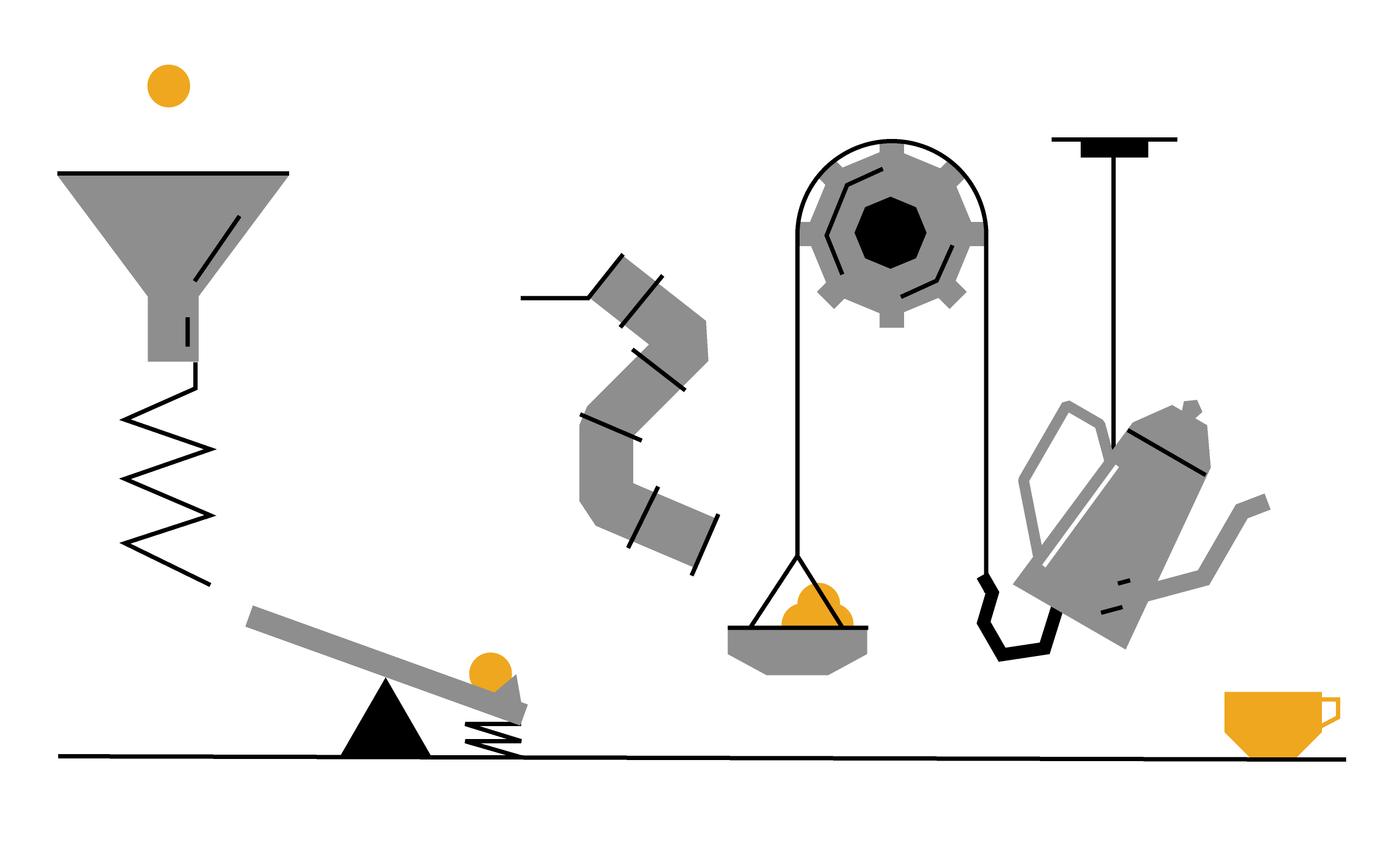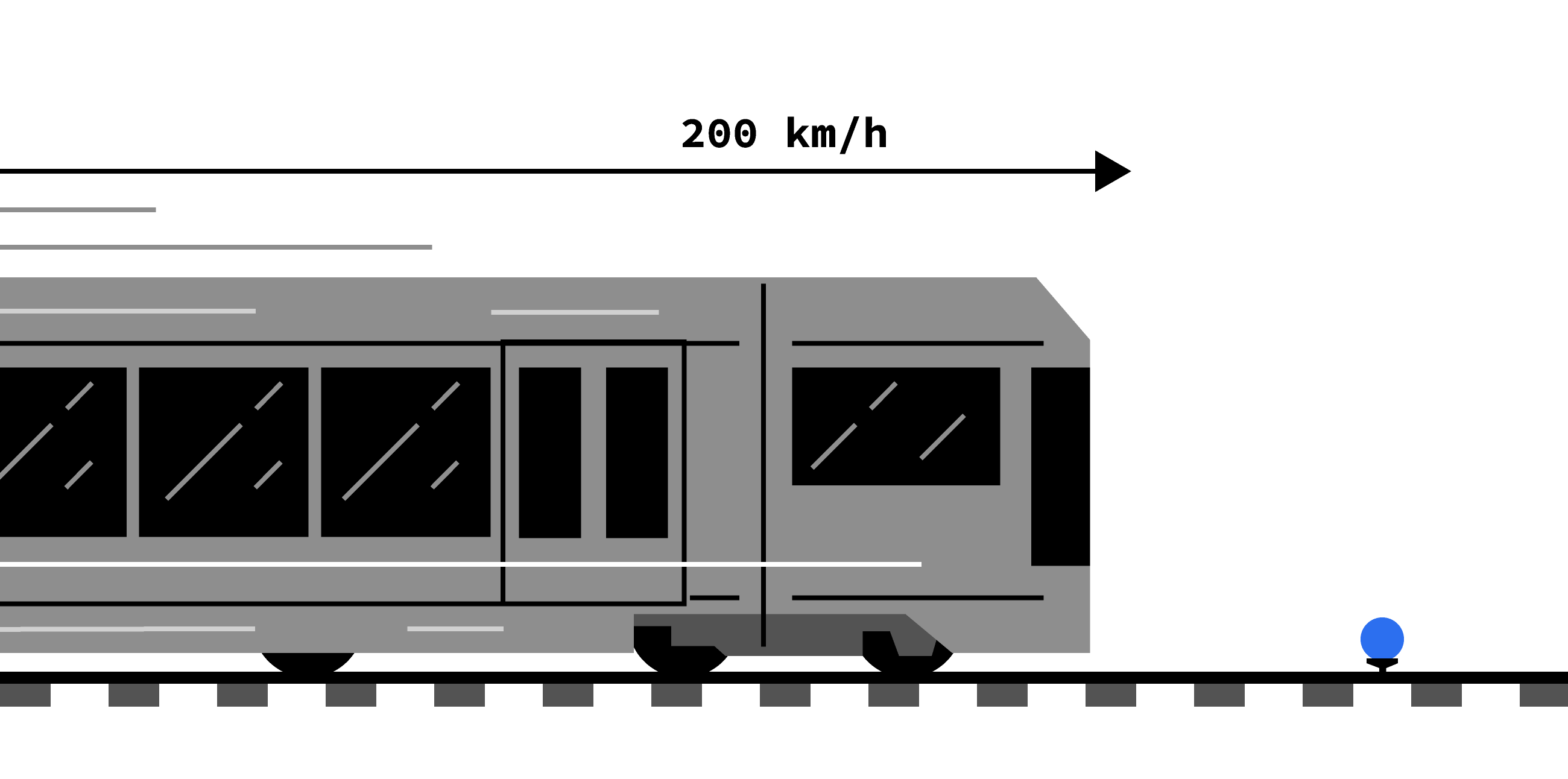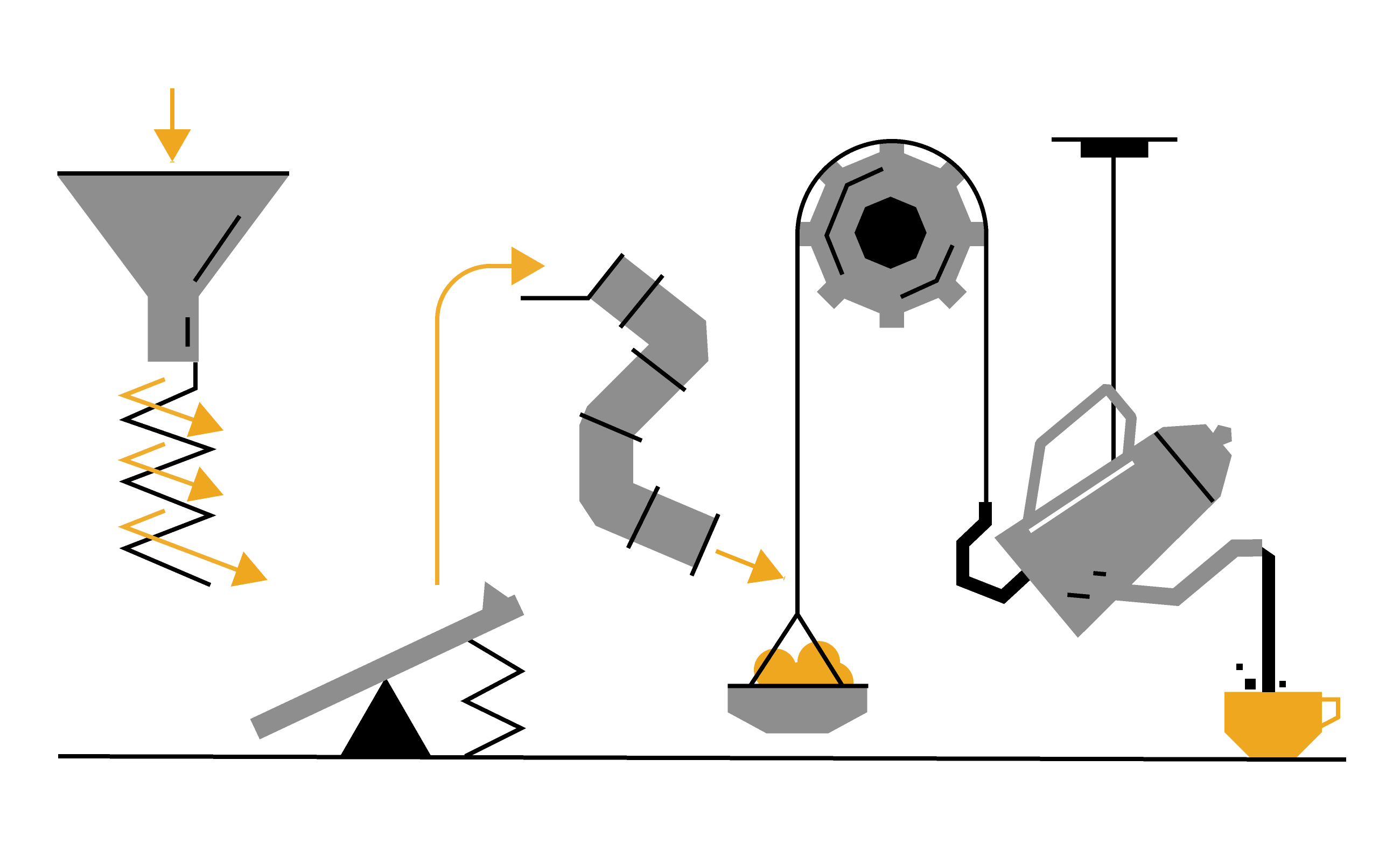### Scientific Thinking

We live in an exciting time!

Science is part of our culture more today than ever before. You can stream self-landing rocket missions on YouTube, and advanced scientific topics like black holes, quantum computing, and epidemiology have made their way into the headlines.But if you want to build the insight needed to make predictions and build new things, a thousand years of scientific principles stand in your way.

The puzzles in this course will start to bridge that gap.

# Nature is a Puzzle

We've all had one of those satisfying "Aha!" moments while poring over a crossword puzzle, deducing the right arrangement of Sudoku numbers, or finding the key piece at the crux of a jigsaw puzzle.Puzzles are fun because they present a challenge for your brain — they all have a simple structure and a clear set of rules for solving them. But in this course, we won't be solving puzzles involving general knowledge, numerical patterns, or image reconstruction.

We'll be tackling puzzles in the natural world.

# Nature is a Puzzle

To solve a puzzle, you have to know its rules, and when the puzzle is nature, its rules are scientific principles.

The physical sciences are the study of these rules, whether it is in the form of chemistry, biology, or physics.

Throughout this course, we'll unwrap the basic building blocks of the natural world, helping you understand motion, forces, energy, heat, light, and time.Everything follows these rules. The world is beautifully connected by the laws of physics that you'll find in this course.

# Nature is a Puzzle

There's no better way to see the power of scientific principles than to use them.

To kick things off, we're going to think about a peculiar problem:

$\\[0.5em]$For reasons left unspecified, a bouncy ball sits on a stand as a commuter train barrels toward it.

After the train collides with the ball, how fast will the ball be moving?To get started, let's think about how balls bounce.

# Nature is a Puzzle

Bouncy balls are nice because their motion is simple. It can be described with the following rule:

Rule: When a bouncy ball collides with a much heavier object that's at rest, it bounces off at the same speed in the opposite direction.

If you don't have a bouncy ball lying around, you can test this out by dragging the ball around and dropping it.

If you throw the bouncy ball at a wall with a speed of $\SI[per-mode=symbol]{2}{\meter\per\second},$ what will its speed be immediately after it bounces?

# Nature is a Puzzle

The first rule might not seem to get us any closer to thinking about the train. After all, the train is moving, not the ball.$\\[-1em]$But that's because we're looking at things from a particular perspective — the one where the train is moving.

This leads us to our second important rule:

Rule: Nature doesn't depend on how we look at it. A physical process is the same no matter what perspective we use to think about it.

Whether we think of it as a $\SI{200}{km/hr}$ train that collides with a ball at rest, or a ball moving at $\SI{200}{km/hr}$ that collides with a train at rest, the ball and train will end up with the same relative speed.

# Nature is a Puzzle

Sometimes, all you need is a little change in perspective to turn a hard problem into an easier problem that you know how to solve.

See if you can do that here:$\\[-1em]$A train is barreling down the tracks at $\SI{200}{\kilo\meter/hr}$ toward our bouncy ball that sits on a stand. After the ball and the train collide, how fast will the ball be moving?

Hint: You'll have to use the two rules together!

# Nature is a Puzzle

As it turns out, this problem is much simpler when we think about it from the perspective of the train!

To a person on the train, the ball approaches the stationary train at $\SI{200}{km/hr}.$ Since the train is much heavier than the ball, the first rule tells us that the ball will bounce back at the same speed it approached, $\SI{200}{km/hr}.$

However, the second rule tells us that the outcome is the same no matter how we look at the collision. In this case, the outcome is the relative velocity of the train and the bouncy ball.

Since the train is itself moving at $\SI{200}{km/hr},$ we need to add its velocity back in to find the final speed of the ball according to a bystander on the side of the tracks.

So, the ball bounces away at

$\overbrace{\SI{200}{km/hr}}^\text{Speed of ball to train} + \overbrace{\SI{200}{km/hr}}^\text{Speed of train to bystander} = \overbrace{\SI{400}{km/hr}}^\text{Speed of ball to bystander}.$

The freedom to switch perspectives is a powerful tool for thinking about the world, commonly known as the principle of relativity, and is the foundations for Einstein's breakthroughs.

# Nature is a Puzzle

The thinking involved in solving puzzles is a unique blend of following rules with imaginative association.

Only by combining both can you see a pattern or a twist that the puzzle conceals. If all goes well, you're able to use that hidden insight to form new information and ideas.The process will be exactly the same with physics: we'll bring the rules, you bring the imagination.

# Nature is a Puzzle

×